# Solving triangles with inequalities

## Basic Properties

In any triangle we can find the following to be true:

(1) The length of each side is less than the sum of the lengths of the other two sides, and greater than the difference between these lengths.

(2) Sides that are not equal are located opposite angles that are not equal, so that the longest side lies opposite the angle with the biggest measure and the shortest side lies opposite the angle with the smallest measure.

In the picture below, points D, E and F represent the vertices of triangle DEF. Since the shortest distance between two points is a straight line, DF must must be less than DE + EF. In other words, imagine if DF was greater than DE + EF. In that case, DE + EF wouldn't be long enough to reach from D to F at all.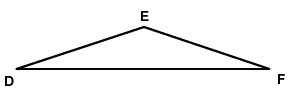To find out if a set of three positive points can represent the lengths of the sides of a given triangle, CHECK that each of the three numbers is less than the sum of the other two numbers.

### Sample A:

In the following picture of triangle ABC, can the set of numbers given represent the lengths of the sides of triangle ABC? Side AB = 4, Side BC = 5 and Side AC = 1.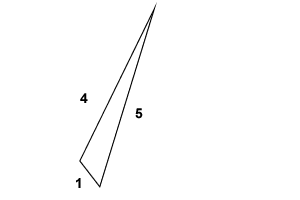Let us check that each of the three given numbers is less than the sum of the other two numbers.

### Solution:

In the above triangle ABC, we can see that although 4 is less than 5 + 1 and 1 is less than 4 + 5, 5 is NOT less than 1 + 4. The answer is no. The set of numbers given does not represent the lengths of the sides of triangle ABC. Imagine if you tried to build this triangle in real life. You have one side that's 5, and the other two sides are exactly 5 when straightened. You can connect them only as two overlapping lines. You need some extra length to make a triangle out of it.

### Sample B:

If 3, 9 and x represent the lengths of the sides of a triangle, how many integer values for x are possible?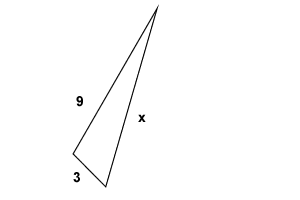Remember that in a triangle, the range of possible lengths of a side depends on the lengths of the other two sides.

### Solution:

The length of a side must be less than the sum of the other two sides. Here, that means x < 9+3, or x < 12.

In addition, remember that the length of a side must be greater than the difference of the other two sides. That means x > 9 - 3, or x > 6.

Therefore we have two limits for the value of x: 6 and 12.

6 < x < 12

Since x is an integer, x can be equal to numbers 7, 8, 9, 10, or 11 (the numbers that lie between 6 and 12 do NOT include 6 and 12). Remember that if we use 6 or 12 we will end up with overlapping straight lines, not a triangle.

### Sample C:

The shortest distance between city X and city Y is 100 miles. The shortest distance between city Y and city Z is 450 miles. What is the shortest distance, in miles, between city X and city Z?

(a) 150 miles
(b) 250 miles
(c) 350 miles
(d) 400 miles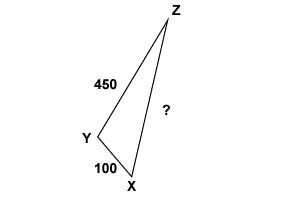We remember that the length of each side of any triangle is greater than the difference between the lengths of the other two sides. If this is true, then XZ is greater than 450 miles - 100 miles or 350 miles.

Since the lengths of each side of a triangle is also less than the sum of the lengths of the other two sides, we can say that XZ is less than 450 miles + 100 miles or 550 miles.

We can conclude that XZ must be between 350 miles and 550 miles. The only answer that makes sense in the choices provided is choice (d) or 400 miles.

## Angles in a Triangle

In a triangle, when two angles have the same measure, their opposite sides also have the same length. At the same time, angles that are NOT equal in measure have opposite sides that do NOT have the same length. I should also say that the angle with the largest measure is opposite the longest side.

### Sample D:

The degree measure of angle A of isosceles triangle ABC is 30 degrees. Sides ABD and AC are equal. Find the shortest side of triangle ABC.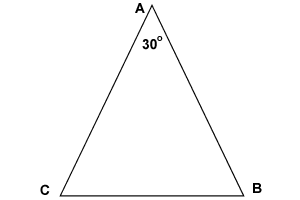### Solution:

We must find the degree measure of the other two angles of triangle ABC. Since the measure of angle A = 30 degrees, then it follows that the other two angles must be equal and add up to 150 degrees. That means they are each 75 degrees. That means the side opposite angle A (side BC) is the smallest side.

Provided by Mr. Feliz